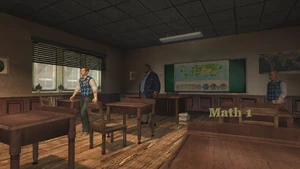This article features content exclusive to the Scholarship and Anniversary editions of Bully. This feature is not available on the original version of the game.
MathData
Teacher Mr. Hattrick
Day Available Day 5
(1pm-3:30pm)
Scholarship Only? Yes
Location Main Building: Top floor, left side from Miss Danvers' office, right next to a locked door that provides roof access
Classmates Bryce
Sheldon
Beatrice

Math is a type of minigame class taught at Bullworth Academy that is exclusive to the Scholarship Edition and Anniversary Edition versions of Bully. It is taught by Mr. Hattrick. The Math minigame involves a timer to answer questions of the following type:

• Mathematics - answer a basic math question
• Which is slowest/fastest - 4 items are pictured, select the slowest/fastest object
• Which is shortest/tallest - 4 items are pictured, select the shortest/tallest object
• Which is smallest/biggest - 4 items are pictured, select the smallest/biggest object
• How many triangles/circles/squares - Count the number of individual shapes

Math 1 Math 2 Math 3 Math 4 Math 5
Reward Genius Hat Math Shirt Shut Your Pi Hole Shirt Hip 2 B Squared Shirt Nerd Outfit
Abacus trophy
Answers 3 + 4 = 7 Smallest: Watermelon 1kg = 1000g Fastest: Airplane 13 + 8 + 2 = 23
50 = 5 + 45 Fastest: Sloth 36 = 6 x 6 Lowest: 0.09 Lowest: 2234
6 x 6 = 36 Triangles: 3 Tallest: Cathedral Triangles: 7 Shortest: Dog house
Triangles: 2 1500 = 30 x 50 0.5 = 1/2 49 = 57 - 8 2 = 9 - 7
Smallest: Mosquito 18 - ? = 5: 13 360 = 180 x 2 0.625kg = 625g 689g = 0.689kg
12 = 20 - 8 Tallest: Eiffel Tower Smallest: Pizza Circles: 8 Squares: 8
Biggest: Planet 100 = 25 x 4 Slowest: Snail 0 = 0 x 125 ? + 6 - 3 = 11: 8
Lowest: 203 2 = 9 - 7 16 + 10 = 26 Biggest: Building Fastest: Airplane
8 + 3 = 11 Biggest: Cathedral 2 + 9 = 11 6 + 8 + ? = 17 = 3 Triangles: 4
1/2 = 0.5 Highest: 771 400 = 20 x 20 Fastest: Fly Highest: 33.8
Slowest: Sloth Shortest: Bird house Smallest: Snail 1/4 = 0.25 255 = 5 x 51
120 = 3 x 40 Slowest: Turtle Biggest: Planet 13+8+2=23 0.75 = 3/4
7 - 5 = 2 1/4 = 0.25 10 - 10 + 7 = 7 Fastest: Rocket Biggest: Bridge
Fastest: Truck 8 + 2 = 10 Lowest: 2234 Shortest: Birdhouse Fastest: Airplane
99 = 11 x 9 Triangles: 2 6 + 5 = 11 15 - ? = 6 = 9 1.25 = 5/4
12 + ? = 21: 9 144 = 12 x 12 Triangles: 7 Triangles: 3 Slowest: Pyramid
35 = 7 x 5 Shortest: Bird house 6/18 = 1/3 Slowest: Turtle 12 + 6 - 11 = 7
Fastest: Rocket 55 = 1 x 55 1g = 0.001kg Squares: 7 3.14 = 31.4 x 0.1
Squares: 5 13 - ? = 5: 8 17 - 11 = 6 3000 = 50 x 60 Triangles: 7
0.5 x 34 = 17 Circles: 6 2 + 7 + 2 = 11 1 + 4 - 2 = 3 7 + ? + 3 = 17: 7
15 - 6 = 9 Fastest: Airplane Smallest: Whale Smallest: Key Fastest: Rocket
Fastest: F1 car 0.75 = 3/4 Fastest: Whale 17 + 8 = 25 Shortest: Headstone
0.5 = 1/2 15 = 3 x 5 190 - ? = 30: 160 1200 = 20 x 60 Biggest: Cathedral
Tallest: Pyramids Lowest: 677 Squares: 7 48500g = 48.5kg 1/8 = 0.125
Highest: 79 Biggest: Truck 13 + 8 + 2 = 23
7 + 3 = 10
24 = 12 x 2

## Trivia

• The fourth reward, the Hip 2 be Square t-shirt, is a reference of the Huey Lewis and the News 1986 song Hip to be Square.
• Similar to English class and Geography class, Jimmy will spin around in his seat, before class starts. This happens in the Wii version and the Xbox 360 version as a glitch. It is more likely to happen in English class, though. This glitch was fixed in the Anniversary Edition
• This subject is known as Maths in the UK and Australia. Despite this, in both Canis Canem Edit and the PAL release of Scholarship Edition, the classroom is labeled "Math" as in the US, and the Scholarship Edition minigame is also called Math. The American name for the subject is most likely used because the game takes place in the US.
• The Math classroom uses the same interior as the English and Geography classrooms.
• Mr. Hattrick still teaches this class after he is fired (similar to Gym class, after Mr. Burton was also fired).